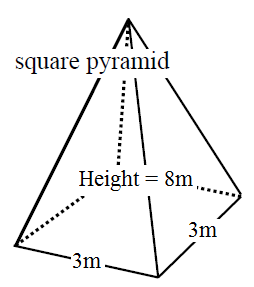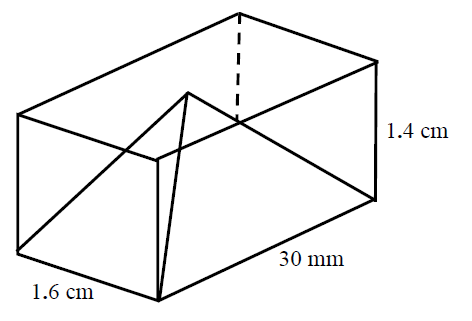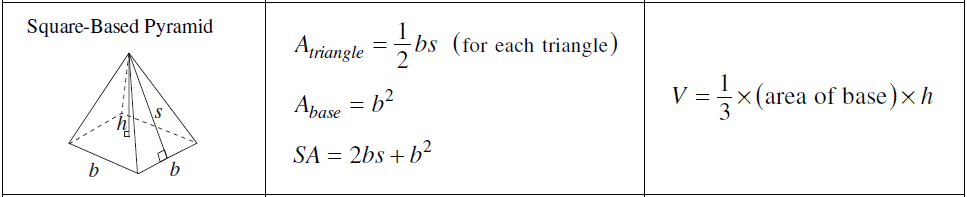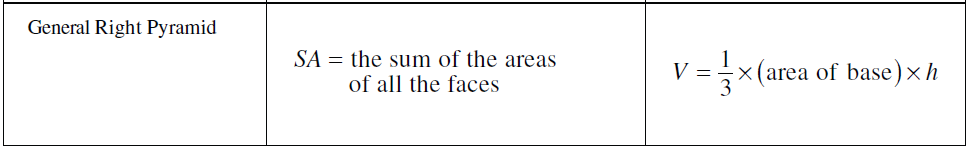# Surface area and volume of pyramids

0/0
0/2
##### Examples
###### Lessons
1. Find the surface area and the volume of the following pyramids:

1.2.###### Free to Join!
StudyPug is a learning help platform covering math and science from grade 4 all the way to second year university. Our video tutorials, unlimited practice problems, and step-by-step explanations provide you or your child with all the help you need to master concepts. On top of that, it's fun - with achievements, customizable avatars, and awards to keep you motivated.
• #### Easily See Your ProgressWe track the progress you've made on a topic so you know what you've done. From the course view you can easily see what topics have what and the progress you've made on them. Fill the rings to completely master that section or mouse over the icon to see more details.
• #### Make Use of Our Learning Aids###### Practice Accuracy

See how well your practice sessions are going over time.

Stay on track with our daily recommendations.

• #### Earn Achievements as You LearnMake the most of your time as you use StudyPug to help you achieve your goals. Earn fun little badges the more you watch, practice, and use our service.
• #### Create and Customize Your AvatarPlay with our fun little avatar builder to create and customize your own avatar on StudyPug. Choose your face, eye colour, hair colour and style, and background. Unlock more options the more you use StudyPug.
###### Topic Notes
The surface area of a pyramid basically contains four triangles and a rectangle as its base. Therefore, if you know how to find the area of triangles and rectangles, we can calculate the surface area of pyramids quite easily. Also, there is a formula for calculating the volume of pyramid too. We will also look at some composite solid questions in this lesson.

## How to find the surface area of a pyramid

The surface area of a $3D$ shape tell us the areas of all the different sides of the shape summed together. In this chapter, we're learning about the surface area and volumes of pyramids. There's many different pyramids such as a square pyramid or a rectangular pyramid. So how do we find the surface area of a pyramid? There are actually formulas to help you find this.

### Surface area formula

For a general right pyramid whose faces are all the same, the surface area = the sum of all the areas of the pyramid's faces.

For a square-based pyramid, you'll need to calculate the area of the triangle using: $\frac{1}{2}bs$ (for each triangle that you're working with), then find the area of the base. This means your formula will be $SA = 2bs + b^{2}$

Essentially, for both of these formulas, you're just finding all the areas of the different sides of a pyramid and then adding it together to get the total surface area. You can imagine the net of a 3D shape spread out, and having to calculate each of the sections of the net's area separately.

## how to find the volume of a pyramid

The volume tells you how much space an object takes up. For a pyramid, you can use the below formula to help you find the answer.

### Formula for volume

Volume of a pyramid = $\frac{1}{3}$ x (area of base) x $h$

## Examples problems

Question:

Find the surface area and the volume

Solution:

First, we are looking for the surface area (SA). We will have to look for the area of the base and the four triangular sides. For the triangular sides, we are missing the height of the triangle. So, let's first look for the height. We can do this with the help of the pythagorean theorem:

$c^{2} = a^{2} + b^{2}$

$s = 8^{2} + 1.5^{2}$

$s = 8.14m$

Area of a triangular side:

$A = \frac{B \times h}{2}$

$A = \frac{3 \times 8.14}{2} = 12.21m^{2}$

There are four sides, so:

$12.21m^{2} \times 4 = 48.84m^{2}$

Now look for the area of the base:

Area of base = $(3)(3) = 9m^{2}$

Add the triangular sides and base together:

SA = $48.84m^{2}+9m^{2} = 57.84m^{2}$

Now, look for the volume of a square pyramid:

$V=\frac{1}{3} \times base.area \times height$

$V=\frac{1}{3} \times (3 \times 3) \times 8$

$V = 24m^{3}$

Question 2:

Find the surface area and the volume

Solution:

We're going to start with looking for the Surface area(SA). Before that, we need to convert unequal units and gather the required information that are not given to us in the graph.

First, we want all numbers in centimeter. So,

$30mm = 3cm$

Then, we need to look for the height of the triangular sides. We have two triangles in different sizes. So we need to look for $s_{1}$ and $s_{2}$. From the graph, we have the following information

Now, calculate $s_{1}$

$c^{2} = a^{2} + b^{2}$

$s_{1} = 1.5^{2} + 1.4^{2}$

$s_{2} = 2.05cm$

Now, calculate $s_{2}$

$c^{2} = a^{2} + b^{2}$

$s_{1} = 0.8^{2} + 1.4^{2}$

$s_{2} = 1.61cm$

Now, look for the surface area

SA of base = $(1.6)(3)$=$4.8cm^{2}$

Look for the area of the sides. We have sides with different sizes. Sides on left and right are identical to each other. It's the same for sides on the front and the back.

Now, calculate the front and back

$F + B = \frac{1.6 \times 2.05}{2} \times 2$

$F + B = 3.28cm^{2}$

Now, calculate the left and right

$L + R = \frac{3 \times 1.61}{2} \times 2$

$L + R = 4.83cm^{2}$

Add the triangular sides and base together:

$SA=3.28cm^{2}+4.83cm^{2}+4.8cm^{2}=12.91cm^{2}$

Now, look for the volume:

$V=\frac{1}{3} \times base.area \times height$

$V=\frac{1}{3} \times (3\times 1.6) \times 1.4$

$V=2.24cm^{3}$

Feel free to explore the volume of a triangular pyramid further with this online volume calculator. If you're ready to move on, learn about the surface area and volume of other shapes and try out some volume word problems.